## Engineering Mechanics Viva Questions

#### Introduction

1. What is Mechanics?
Ans: It is defined as a branch of physical science that is concerned with the study of various forces acting on a body when the body is in a state of rest or in motion. Mechanics is subdivided into three Branches; Mechanics of rigid bodies, Mechanics of deformable bodies and Mechanics of Fluids.In Our course, E. M is restricted to the study of mechanics of rigid bodies only

2. Which are the different types of Mechanics?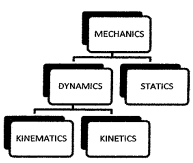Ans: STATICS: it is a branch of E. M which deals with various forces acting on a body at rest. DYNAMICS: It is a branch of E. M which deals with the study of motion.
KINEMATICS: It is the study of the motion of a body without consideration of forces which cause motion, It
analyses geometry of motion KINETICS: Which relates forces acting on the body to the motion of the body. and shape

3. What is RIGID BODY?
Ans: On the application of force, the body does not change its size

4.What is Particle?
Ans: Considerable mass but Negligible Dimension

5. Newton’s First Law of Motion
Ans: Every Body continues in its state of rest or in uniform motion in an in a straight line unless it is acted upon by an unbalanced force

6. Newton’s Second Law of Motion
Ans: The rate of change of momentum is directly proportional to the impressed force and takes place in the direction of Force.

7. Newton’s Third Law of Motion
Ans: For every action, there is the equal and opposite reaction

#### Module-1

1. What is FORCE
Ans. Force is defined as an agency that tends to change the state of rest or of uniform motion of a Body. Force is a Vector Quantity.

2. Characteristics of a Force?
Ans. 1) Magnitude
2) Direction
3) Point Of Application
4) Line of Action: Line along which force acts.

3. Unit of Force: S1 unit of force is Newton (N) or Kilo Newton.
Ans. 1 Newton force is defined as the force required to produce an acceleration Of 1 m/s².

4. Explain different types of Forces?
Ans. 1) Coplanar Forces: All forces exist in a single plane.

• Concurrent All the forces Line of action passes through a single point
• Parallel: Forces which are parallel to each other General Force system: Forces which are neither parallel nor concurrent.
• General Force System: Forces that are neither parallel nor concurrent.

2) Non-Coplanar force: Forces lies in more than one plane

5. What is the External Effect of a Force
Ans. Force when applied on a body, may change or tend to change the state of rest or in state of motion of a body.

6. What is Internal Effect of Force:
Ans: Under the application of forces on a body internal stresses get set up in the body due to which the body deforms

7. What is System of Force?
Ans: It is a group of forces under consideration

8. What is the Law of Parallelogram?
Ans: If Two forces acting at a point can be represented in magnitude and direction by two adjacent sides of a parallelogram, drawn from one of its angular points, they’re resultant by the diagonal of the parallelogram passing through that angular point.

9. What is the Law of Triangle?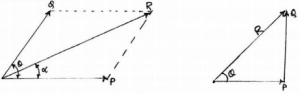Ans: If Two forces acting at a point are represented in magnitude and direction by the two sides of a triangle taken in order their resultant is represented by the third side taken on opposite order.

10. What is RESULTANT
Ans: It is the single force that replaces all the forces acting on a system.

11. What is the Resolution of force
Ans: The way of representing a single force into a number of forces without changing its effect on the body.

12. What is Equilibrant
Ans: The equilibrant of two or more forces acting on a body is a single force which when acting with other forces keeps the body in equilibrium.

13. What is the difference between a resultant force and an equilibrant force?
Ans: Resultant force makes the object move whereas equilibrant force keeps it in equilibrium

14. State Superposition Principle?
Ans: If more than one force acts on an object, the combined effect due to all the forces is the vector addition of all the individual’s effects.

15. Principle of Transmissibility of force?
Ans: Force is a sliding vector that continues to act along its line of action and therefore makes no change it acts from a different point on its line of action on rigid body

16. Define Couple?
Ans: Two non-collinear parallel forces having the same magnitude but opposite direction forms a couple. The couple is a free vector

17. Define Moment?
Ans: Turning effect of force produces Moment M-FX d
Where M=Moment in N/m F= Force
de Perpendicular distance in Metres

18. Distinguish Between A Couple and Moment?
Ans: Moment represented turning effect of a force whereas couple consists of two equal and opposite forces separated by some distance. The moment of force varies from point to point. The couple is the same about any point in the plane

#### Module-2

1. What is Equilibrium
Ans: If the resultant of the force system is ZERO, syster\m is said to be in Equilibrium.

2. Condition of Equilibrium
Ans: EF=0&EF-0(Body can not translate) EM-0(Body does not Rotate)

3. What is Lami’s Theorem?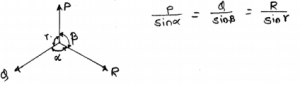Ans: It is applicable for only 3 concurrent forces. if 3 concurrent forces are in equilibrium then the magnitude of each force is proportional to the sine of the angle between the other two forces in the system.

4. Explain FREE BOGY DIAGRAM (FBD)?
Ans: It is a diagram of the body under consideration showing all forces, active and reactive acting on the body F80 gives a simple idea about the system of forces acting on a body. We are isolating the body From surrounding and then shows all forces acting on it.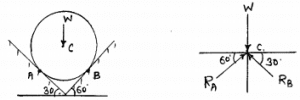Ans: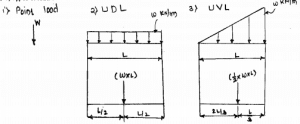6. What is a BEAM
Ans: The beam is a structural member subjected to transverse loading only.

7. What is MEMBER
Ans: A two force straight member is always in tension or compression

8. When the moment of a force is zero?
Ans: Moment of a force about a point is zero if its line of action passes through that point

9. Explain Compound Beam?
Ans: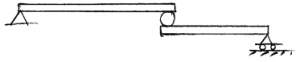10. What is Truss?
Ans: It is designed to support the load and usually stationary member of a truss is a 2 force member

11.  ASSUMPTIONS IN TRUSSES?
Ans:

• Members are straight and rigid.
• Force will act at joint only
• The weight of a member is neglected
• The Joint is made up of frictionless pins

12. Method of Analysis
Ans:

•  Method of joints: It forms a concurrent force system. Max 2 unknowns.
•  Method of section: Maximum
•  Unknowns will be cut.

#### Module-4

1. For constant velocity acceleration of a body is zero

2. Distance-displacements when the body moves in only one direction of motion

3. For Maximum or minimum velocity acceleration of a body is zero

4. Slope of DV/DT gives acceleration

5. Slope of da/dt Gives – Jerk

6. Area under v-t diagram gives = change in position

7. Area under a-t diagram gives the change in velocity.

#### Module-5

1. What is kinematics?
Ans: The branch of mechanics is concerned with the motion of objects without reference to the forces which cause the motion.

2. Difference between kinetics and kinematics?
Ans: Kinematics is the study of an object moving without the involvement of force while the kinetics is the study object’s movement with the involvement of force.

3. What are the different types of rigid body motions?
Ans:

• Translational
• Rotation about a fixed axis
• General plane motion
• Motion about a fixed point
• General Motion

4. What is translation motion and state its type?
Ans: In translation motion, all the particles forming the body travel along parallel paths. Also, the orientation of the body does not change during motion. Classified as: 1) Rectilinear Translation and 2) Curvilinear Translation.

5. Define Rectilinear and Curvilinear Translation.
Ans: Rectilinear Translation: All the particles travel along straight parallel paths.
Curvilinear Translation: All the particles travel along curved parallel paths.

6. Define Axis of rotation
Ans: The straight line through all fixed points of a rotating rigid body around which all other points of the body move in circles

7. Define :
1. Angular Displacement: The change in the angular position of the body during its motion is known as the angular displacement of the body.
2. Angular Velocity: The rate of change of angular position wrt time is the angular velocity of the rotating body.
3. Angular Acceleration: The rate of change of angular velocity wrt time is the angular acceleration of the rotating body.

8. State different types of Rotation Motion about a fixed axis.
Ans: 1) Uniform angular velocity motion
2) Uniform angular acceleration motion
3) Variable angular acceleration motion

9.What is the instantaneous Centre Method?
Ans: Instantaneous centre is defined as the point about which the G.P. the body rotates at the given instant.

10. What is a three-dimensional motion?
Ans: In this type of rigid body motion, the body rotates about a fixed point, but the axis of rotation passing through the fixed point is not stationary as its direction keeps on changing.

#### Module-6

1. What is Friction
Ans: It May be defined as a resistive force acting opposite direction to motion. Frictional force (F) always acts along surfaces, the unit of friction is N.

2. VERGE OF MOTION or IMPENDING MOTION
Ans: For a body, there will be the value of Frictional force up to and body is in equilibrium. Maximum Frictional force is called LIMITING FRICTION. The state of the body is called impending motion.

3. Angle of friction
Ans: The angle between Normal reaction and the resultant reaction tan u

4. Cone of friction
Ans: It is an imaginary cone generated by revolving resultant reaction about the normal reaction

5. LAW OF FRICTION
Ans: The frictional force is always opposite to the direction of motion, Friction is independent of the area of contact Ratio of limiting Frictional force F and normal reaction N is constant.

6. Significance of Wedges?
Ans: Wedges are generally used to lift heavy machines blocks

7. What is Centre of Gravity
Ans: It is the point through which the whole weight of a body is supposed to act.

8. What is Centroid
Ans: A term used for CG of all plane geometrical figures.

9. What is  Avis of symmetry
Ans: Fine which divides a figure into two equal parts, such that each part is the mirror image of other

1. Spring force: Force in spring Is variable as it is proportional to deformation X and directed towards the neutral position.

2. Impulse: Is the Product of force and duration for which its acts.

3. Momentum: Product of mass Velocity

4. Impulse Momentum Equation: The momentum of a body changes and the change in momentum is equal to the impulse produced by force.

5. Impact: The collision of two bodies takes place during the very short interval

6. Central impact: Mass centres of both bodies are in the line with the impact

7. Oblique Impact: Mass centres of both bodies are NOT in line with the impact